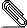# Re: [PATCH 1/2] usb: serial: option: Add the BroadMobi BM818 card

```On 05/08/2019 12:47, Johan Hovold wrote:
> On Wed, Jul 24, 2019 at 07:52:26AM -0700, Angus Ainslie (Purism) wrote:
>> From: Bob Ham <bob....@puri.sm>
>>
>> Add a VID:PID for the BroadModi BM818 M.2 card
>
> Would you mind posting the output of usb-devices (or lsusb -v) for this
> device?```
```
T:  Bus=01 Lev=03 Prnt=40 Port=03 Cnt=01 Dev#= 44 Spd=480 MxCh= 0
D:  Ver= 2.00 Cls=00(>ifc ) Sub=00 Prot=00 MxPS=64 #Cfgs=  1
P:  Vendor=2020 ProdID=2060 Rev=00.00
S:  Manufacturer=Qualcomm, Incorporated
S:  Product=Qualcomm CDMA Technologies MSM
C:  #Ifs= 5 Cfg#= 1 Atr=e0 MxPwr=500mA
I:  If#=0x0 Alt= 0 #EPs= 2 Cls=ff(vend.) Sub=ff Prot=ff Driver=(none)
I:  If#=0x1 Alt= 0 #EPs= 2 Cls=ff(vend.) Sub=ff Prot=ff Driver=(none)
I:  If#=0x2 Alt= 0 #EPs= 3 Cls=ff(vend.) Sub=ff Prot=ff Driver=(none)
I:  If#=0x3 Alt= 0 #EPs= 3 Cls=ff(vend.) Sub=fe Prot=ff Driver=(none)
I:  If#=0x4 Alt= 0 #EPs= 3 Cls=ff(vend.) Sub=ff Prot=ff Driver=(none)

```signature.asc
Description: OpenPGP digital signature